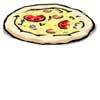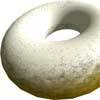#### You may also like### Pizza Portions

My friends and I love pizza. Can you help us share these pizzas equally?### Doughnut

How can you cut a doughnut into 8 equal pieces with only three cuts of a knife?### Pies

Grandma found her pie balanced on the scale with two weights and a quarter of a pie. So how heavy was each pie?

# Fraction Fascination

##### Age 7 to 11Challenge Level

We had some good solutions come in for this challenge.

Sophie and Emma, Keenan and Dylan from Crossacres Primary School wrote:

The smaller square: We traced the shape on tracing paper. Then we cut the triangle shapes out. We found out that the two big triangles fitted to make half (we had to flip one over). Because there were two of them, we halved a half which makes $\frac{1}{4}$.

Then we saw that the small triangle was half of a quarter which is $\frac{1}{8}$.

Then we turned all of the fractions into eighths and added them together and got $\frac{5}{8}$.

This means that the last triangle (the big one) is equal to $\frac{3}{8}$ because $\frac{5}{8}$ + $\frac{3}{8}$ = 1 whole.

and

You will need scissors and more than one copy of the shapes. First we cut out the four triangles that make up the small square. Then we took the two right-angled triangles so that they made an oblong. We put the oblong on top of another copy of the square.The two oblongs covered a half of the square, that makes them a quarter each. This means that the large triangle and small triangle together make up a half too. We then made a square using two of the small triangles. When we put this square on the original square we could see that it equals a quarter. That means that the small triangle is equal to one eighth because that is half of a quarter. The large triangle is equal to three eighths. Work this out by adding one quarter + one quarter + one eighth = five eighths and then to make 1 whole it is three eighths (the largest triangle).

When we looked at the second big square straight away we could see that it was made up of four of the smaller squares. That means that each of the fractions we first worked out would be four times smaller. We separated the large square into the four smaller squares and wrote down what each of the triangles was now worth. To work out the size of the rhombus we worked out
$\frac{1}{16}$ + $\frac{1}{16}$ + $\frac{1}{16}$ + $\frac{1}{16}$ = $\frac{4}{16}$.

Then we simplified the fraction to $\frac{1}{4}$.

Then we checked our answer by using four cut out triangles we placed them on the square and they covered one quarter of the shape.

Esme from St John's CEP School sent in the following:

For the first picture I drew two lines to make quarters on the picture.  In the top half one triangle is half of the half (to make the fractions easy to understand I changed them into eighths) which is two eighths and as the top triangle is the same as the left hand side triangle you know that the left hand side triangle is also two eighths.
In the bottom right hand corner the triangle is half the quarter so the triangle is one eighth.
Then if you add up all the eighths you get five eighths that means that the remaining centre triangle is three eighths.

For the second picture I split it into sixteenths but I calculated it in to thirty-twoths so I could work out the answer more easily.
In the top right hand corner triangle it was half of two sixteenths which (in thirty-twoths) is two thirty-twoths.  That is the same as each corner and the quarters of the big middle shape which means overall the middle shape is eight thirty-twoths.
In the centre of the right and left hand side each side's two sixteenths is one sixteenth of the two sixteenths.  That means that each side triangle is two thirty-twoths.
Then if you add up the triangles you have calculated so far you get twenty thirty-twoths so the remaining shapes have twelve thirty-twoths all together so each of the remaining triangles is three thirty-twoths.

Lydia also from St. John's wrote:

I did this by looking at the bottom line and realising that the central triangle touched halfway, so I drew a vertical line from this point upwards. This then shows that the triangle on the right is half of the rectangle you have just created, therefore the triangle is a quarter of the entire square.
On the left hand side the central triangle's point touches halfway too and I drew the line horizontally across; now the square is in quarters. The top two quarters are split in half by a second triangle which makes that triangle a quarter and together with the triangle on the right a half. Half of one of the smaller squares (bottom left) is filled with a tiny right-angled triangle, and half a quarter is an eighth so the small triangle is one-eighth of the entire square. So now you have one-eighth and a half which makes five eighths. 8 minus 5 equals 3 so the central triangle is three as that is the remaining value!

Bottom left triangle = $\frac{1}{8}$;  Top triangle = $\frac{2}{8}$;  Triangle to the right = $\frac{2}{8}$;   Central triangle = $\frac{3}{8}$

Here is a response from Esther and Tracy from Withernsea Primary School:

First we drew a vertical and horizontal line in order to split the square into quarters. We then looked at the triangle on the left of the square and decided that it was half of the left half of the square. This meant that it was a quarter of the whole square.
The triangle at the top of the square was also a quarter.
The smallest triangle was half the size of these two. Half of a quarter is an eighth.
So, the three triangles were a quarter, a quarter and an eighth.
To calculate the last triangle we turned the denominators into eighths. So, they became $\frac{2}{8}$, $\frac{2}{8}$ and $\frac{1}{8}$. This meant that the central triangle must have been $\frac{3}{8}$ as: $\frac{2}{8}$ + $\frac{2}{8}$ + $\frac{1}{8}$ + $\frac{3}{8}$ = $\frac{8}{8}$.

For the next problem we again divided the square into quarters. Looking at the top left hand square, we decided that the part of the central shape was a quarter of this square. This means that this must be one sixteenth of the whole shape. As there is are four of these triangles, they must take up four sixteenths of the whole square. So the central shape is four sixteenths or one quarter of the whole square.

Sakura, Ema and Wictoria at the VIenna International School in Austria we had sent in the following;

We first labelled the four triangles in size order A, B, C, D. Then we cut the triangles up and noticed that triangles B + C were equal sizes and together measured half the square. Therefore B was $\frac{1}{4}$ and C was $\frac{1}{4}$ of the total area.

Next we split the original diagram into four quadrants. We identified that triangle D was half of a quadrant and therefore equated to $\frac{1}{8}$ of the total area.

We then converted all of our fractions to eighths. We had $\frac{2}{8}$, $\frac{2}{8}$ + $\frac{1}{8}$ = $\frac{5}{8}$. Therefore we knew triangle A would be $\frac{3}{8}$ as the whole would add up to $\frac{8}{8}$.
We checked our answer by cutting the triangles further.

Zach sent in an excellent account of his method of finding solutions. He began by saying:

Start by labelling the triangles. Note that Triangles A, C & D are right angled, whilst Triangle B is isosceles. Triangle A = Triangle C

To read Zach's full solution, see one of the following:Fraction Fascination - Zach.docx or  PDF Fraction Fascination - Zach .pdf

Thank you for these interesting solutions that were sent in. Please keep sending in reports on how you set about doing these challenges.